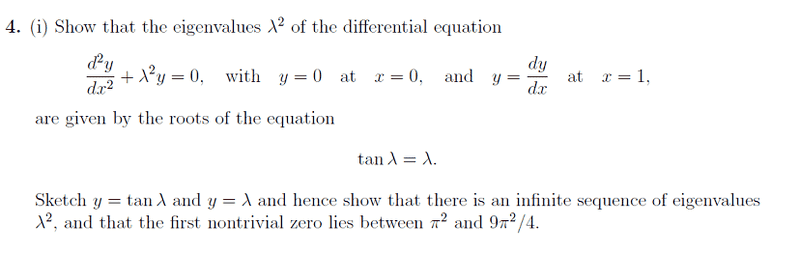# Finding the nontrivial zeros of Tan x = x

• Firepanda

#### FirepandaI can do the first part no problem.

I then drew the graph, am I right in saying there is an infinite sequence because the lines intersect an infinite amount of times, because tan is periodic and has vertical asymptotes?

I have no idea about showing why the first non rivial zero is bounded like that. I would have thought the trivial zero was at the origin, and the first non trivial was in the range of 0<lambda<pi/2.

What am I not understanding here?

Thanks

edit:

oh I think I'm suppose to be looking for solutions in the range of pi and 3pi/2?

oh I think I'm suppose to be looking for solutions in the range of pi and 3pi/2?

...because $\lambda$ is a positive number, so the first non-trivial zero of the equation will be in the next quadrant where $\tan \lambda$ is positive, and you're after $\lambda^{2}$.

[EDIT: On thinking about this a little more, we could also go in the negative direction, since $\tan \lambda$ and $\lambda$ both have odd symmetry and we're looking for solutions for $\lambda^{2}$... But it is easier to think about going in the positive direction.]

BTW, I think the problem-poser means the first non-trivial zero of the function $(\tan \lambda ) - \lambda$ ; that last statement reads a little strangely...

Last edited: